# Mathematics

### Chapter : Linear Equation In Two Variable

#### Examples of Solutions of Pair of Equations

Example: Show graphically that the system of equations x – 4y + 14 = 0 ; 3x + 2y – 14 = 0 is consistent with unique solution.

Solution: The given system of equations is
x – 4y + 14 = 0 ....(1)
⇒ y =When x = 6, y == 5
When x = – 2, y =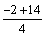= 3
In tabular form

 x 6 −2 y 5 3 Points A B

3x + 2y – 14 = 0 ....(2)

⇒  y =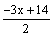When x = 0, y == 7
When x = 4, y =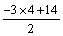= 1
In tabular form

 x 0 4 y 7 1 Points C DThe given equations representing two lines, intersect each other at a unique point (2, 4). Hence, the equations are consistent with unique solution.

Example: Show graphically that the system of equations 2x + 5y = 16 ; 3x +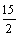y = 24 has infinitely many solutions.

Solution: The given system of equations is
2x + 5y = 16 ....(1)
⇒ y =When x = 3, y == 2
When x = – 2, y == 4
In tabular form

 x −2 3 y 4 2 Points A B

3x +y = 24 ....(1)

⇒ y =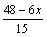....(2)
When x =, y == 3
When x =, y =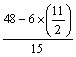= 1
In tabular form

 xy 3 1 Points C DThe lines of two equations are coincident. Coordinates of every point onthis line are the solution. Hence, the given equations are consistent with infinitely many solutions.

Example: Show graphically that the system of equations 2x + 3y = 10, 4x + 6y = 12 has no solution.

Solution: The given equations are 2x + 3y = 10
⇒ 3y = 10 – 2x ⇒ y =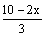When x = – 4, y === 6
When x = 2, y === 2
In tabular form

 x −4 2 y 6 2 Points A B

4x + 6y = 12

⇒ 6y = 12 – 4x
↠ y =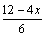When x = – 3, y =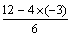= 4
When x = 3, y =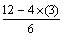== 0
In tabular form

 x −3 3 y 4 0 Points C B

Plot the points A (–4, 6), B(2, 2) and join them to form a line AB. Similarly, plot the points C(–3, 4), D(3, 0) and join them to get a line CD.Clearly, the graphs of the given equations are parallel lines. As they have no common point, there is no common solution. Hence, the given system of equations has no solution.